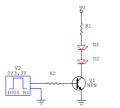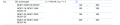# Question about NPN low side switching schematic, finding base resistor.

#### gandoN9

Joined Oct 12, 2020
18First thing i need to do is to find R1 knowing Vcc is 9V and LED current is 20mA.

So i am calculating R1= 9V-3.3V/20mA = 285 ohms, i will choose 330 ohm resistor.

Transistor beta gain is 100

Second task is to find R2 size, which would be in saturation for 3.3V input signal.

So i need to calculate Ic (collector current) Ic= Vcc-Vce/R = 9V-3.3V/330 = 17mA (0.017A)

Then i need base current. Ib = Ic/B(beta) = 0.017A/100 = 0.00017A

And then finally Rb =Vin-Vbe/Ib = 3.3-0.7V/0.00017A =~15k ohms.

Are my calculations for finding Rb right?

Help will be appriciated, thanks!

#### AlbertHall

Joined Jun 4, 2014
12,187
Only if this is a simulated circuit where the gain of the transistor can be precisely specified.

With a real transistor the gain values can have a very wide spread, and further will vary with collector current and collector voltage.
A rule of thumb to make sure the transistor is saturated is to make the base current 10 times the value you calculate like that.

N.B. Your circuit shows two LEDs in series. Is 3.3V twice the expected individual LED voltage?

#### Papabravo

Joined Feb 24, 2006
19,576
NO. The beta of a transistor is for the current gain in an amplifier, and it varies a great deal among transistors of the same type. In practice for a specified beta of 100 it could be anywhere in the range of 50 to 300 for a typical transistor. For a switching application what you wan to do is force the beta to be about 10. So in your example with Ic = 17 ma, you choose Ib = 1.7 ma. The collector current can never be more than 17 ma, limited as it is by the collector resistor and the forward voltage of the LEDs.

Compute R2 to allow the signal generator to supply 1.7 ma @3.3 Volts, and you are done.
Now the actual beta of the transistor is irrelevant.

#### gandoN9

Joined Oct 12, 2020
18
NO. The beta of a transistor is for the current gain in an amplifier, and it varies a great deal among transistors of the same type. In practice for a specified beta of 100 it could be anywhere in the range of 50 to 300 for a typical transistor. For a switching application what you wan to do is force the beta to be about 10. So in your example with Ic = 17 ma, you choose Ib = 1.7 ma. The collector current can never be more than 17 ma, limited as it is by the collector resistor and the forward voltage of the LEDs.

Compute R2 to allow the signal generator to supply 1.7 ma @3.3 Volts, and you are done.
Now the actual beta of the transistor is irrelevant.

I am using BC817 transistor, looking the data sheet:Says the minimum hfe is from 100-600. So i am using beta 100

Do i still need to change something?

#### MrChips

Joined Oct 2, 2009
27,679
Yes. For putting a transistor into full saturation you can ignore the datasheet current gain.
As a rule of thumb, use a current gain = 10.
For collector current = 20mA, use base current = 2mA

Base resistor = 2V / 2mA = 1kΩ

#### Papabravo

Joined Feb 24, 2006
19,576
Yes. A "switching" application for the transistor is fundamentally different from an "amplifier" application. In your "switching" application the transistor is always in one of two states: saturation (fully on) or cutoff (fully off) with a very short duration transition between the two states. In an "amplifier" application the transistor spends all of its time in the linear region and never goes into cutoff or saturation. If an amplifier dis this you would observe clipping and distortion on the output. Generally you don't want an amplifier to do this. In your switching application you make sure the transistor is in saturation by overdriving the transistor and using external components to force the "beta" of the circuit to some small value like 10. It could be 8 or 12 or some other number in that order of magnitude. It just needs to be a small fraction of the beta you would use to design an amplifier that operates in the linear region. In a "switching" application, you are trying to ensure that the transistor spends NO APPRECIABLE AMOUNT of time in the linear region.

•gandoN9

#### dl324

Joined Mar 30, 2015
15,446

•gandoN9

#### gandoN9

Joined Oct 12, 2020
18
So i did new calculations then :

So i am calculating R1= 9V-3.3V/20mA = 285 ohms, i will choose 330 ohm resistor.

Finding R2:

1) Ic= Vcc-Vce/R = 9V-3.3V/330 = 17mA (0.017A)

2) Ib = Ic/B(beta) = 0.017A/10 = 0.0017A = 1.7mA

3) Rb =Vin-Vbe/Ib = 3.3-0.7V/0.0017A = ~1,5k ohm resistor

Is this looking okay now?

#### djsfantasi

Joined Apr 11, 2010
8,661
So i did new calculations then :

So i am calculating R1= 9V-3.3V/20mA = 285 ohms, i will choose 330 ohm resistor.

Finding R2:

1) Ic= Vcc-Vce/R = 9V-3.3V/330 = 17mA (0.017A)

2) Ib = Ic/B(beta) = 0.017A/10 = 0.0017A = 1.7mA

3) Rb =Vin-Vbe/Ib = 3.3-0.7V/0.0017A = ~1,5k ohm resistor

Is this looking okay now?
Are you sure Vce is equal to 3.3V?

#### dl324

Joined Mar 30, 2015
15,446
3) Rb =Vin-Vbe/Ib = 3.3-0.7V/0.0017A = ~1,5k ohm resistor

Is this looking okay now?
Check the first equation.

Equations are easier to read if you use LaTex:
$$R_b = \frac{V_{in}-V_{be}}{I_b}=\frac{3.3V-0.7V}{1.7mA}=1.53k\Omega$$

EDIT: corrected equation and typo.

Last edited:
•gandoN9

#### Papabravo

Joined Feb 24, 2006
19,576
Are you sure Vce is equal to 3.3V?
It is not Vce, it is the Vf of the two LEDs in series. He could use the datasheet value or some extrapolation of it for the lower current. To a first approximation Vce is usually neglected, or estimated at about 0.2 Volts.

•gandoN9

#### djsfantasi

Joined Apr 11, 2010
8,661
It is not Vce, it is the Vf of the two LEDs in series. He could use the datasheet value or some extrapolation of it for the lower current. To a first approximation Vce is usually neglected, or estimated at about 0.2 Volts.
I knew that. I wanted to find out if the person doing the homework did?

•Papabravo and ericgibbs

#### gandoN9

Joined Oct 12, 2020
18

So i am calculating R1= 9V-3.3V/20mA = 285 ohms, i will choose 330 ohm resistor.

Finding R2:

1) Ic= Vcc-Vce/R = 9V-0.2V/330 = 0.026A

2) Ib = Ic/B(beta) = 0.026A/10 = 0.0026A

3) Rb =Vin-Vbe/Ib = 3.3-0.7V/0.0026A = 1000 ohms

Is this looking okay now?

Thank you guys!

#### crutschow

Joined Mar 14, 2008
31,116
Do i still need to change something?
Not if you don't mind a 1V drop from the collector to ground.

But if you want the saturated value of a couple tenths of a volt, then use a Beta value of 10 as recommended here.

#### Papabravo

Joined Feb 24, 2006
19,576
I knew that. I wanted to find out if the person doing the homework did?
I think he might be in overload mode, by substituting one thing for another without realizing that there are multiple things going on.
1. Collector supply voltage, 9 V nominal
2. Collector resistor 285 to 330 Ω
3. Forward voltage drop of 2 LEDs, approximately 3.3 Volts
4. Voltage drop across the Collector Emitter Junction, 0.2 to 0.4 (guessing, without consulting data sheet)

#### BobaMosfet

Joined Jul 1, 2009
2,053
View attachment 220821

First thing i need to do is to find R1 knowing Vcc is 9V and LED current is 20mA.

So i am calculating R1= 9V-3.3V/20mA = 285 ohms, i will choose 330 ohm resistor.

Transistor beta gain is 100

Second task is to find R2 size, which would be in saturation for 3.3V input signal.

So i need to calculate Ic (collector current) Ic= Vcc-Vce/R = 9V-3.3V/330 = 17mA (0.017A)

Then i need base current. Ib = Ic/B(beta) = 0.017A/100 = 0.00017A

And then finally Rb =Vin-Vbe/Ib = 3.3-0.7V/0.00017A =~15k ohms.

Are my calculations for finding Rb right?

Help will be appriciated, thanks!
This book will be invaluable to you:

Title: Understanding Basic Electronics, 1st Ed.
Publisher: The American Radio Relay League
ISBN: 0-87259-398-3

#### Alec_t

Joined Sep 17, 2013
13,228
Forward voltage drop of 2 LEDs, approximately 3.3 Volts
What colour LEDs?